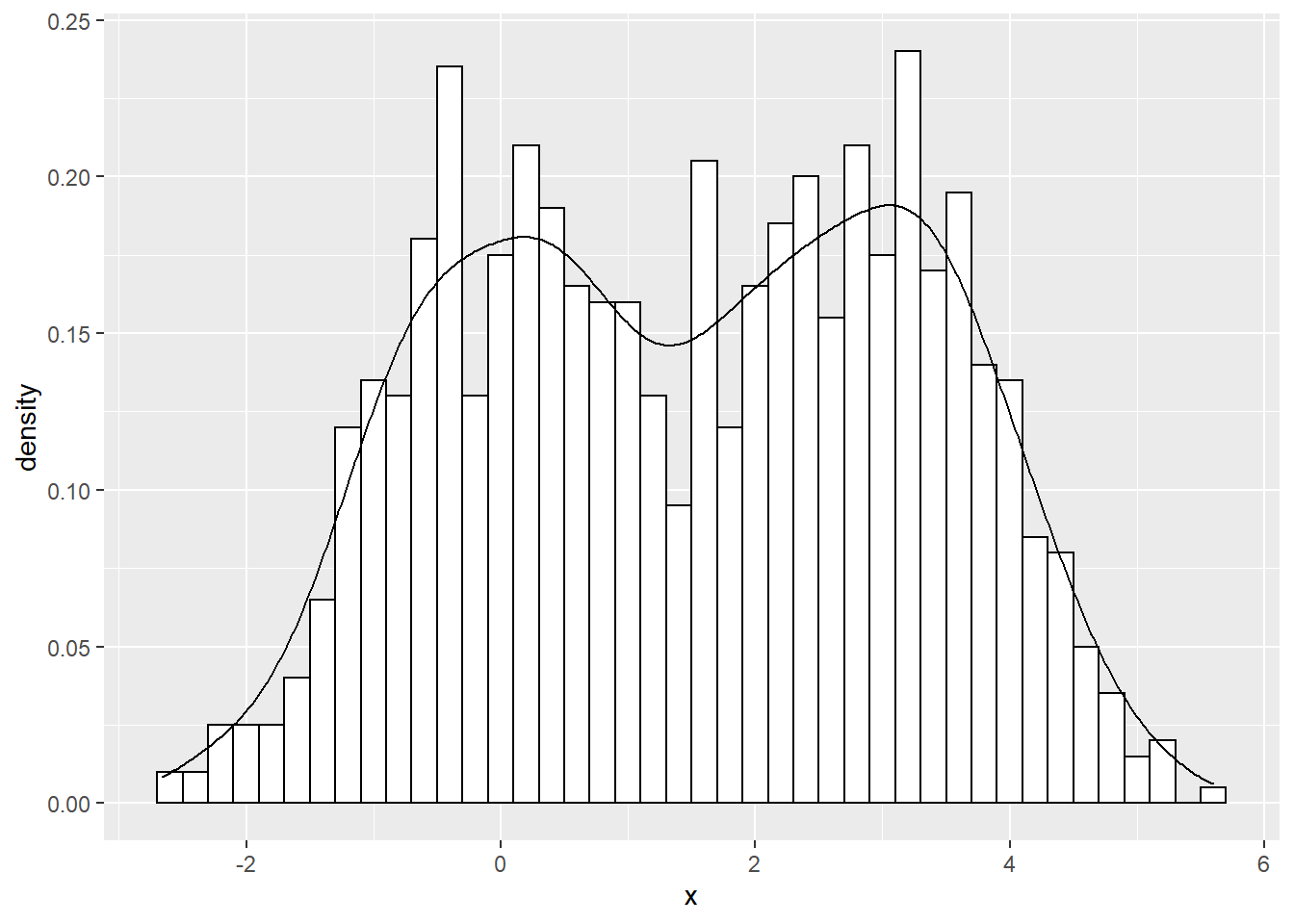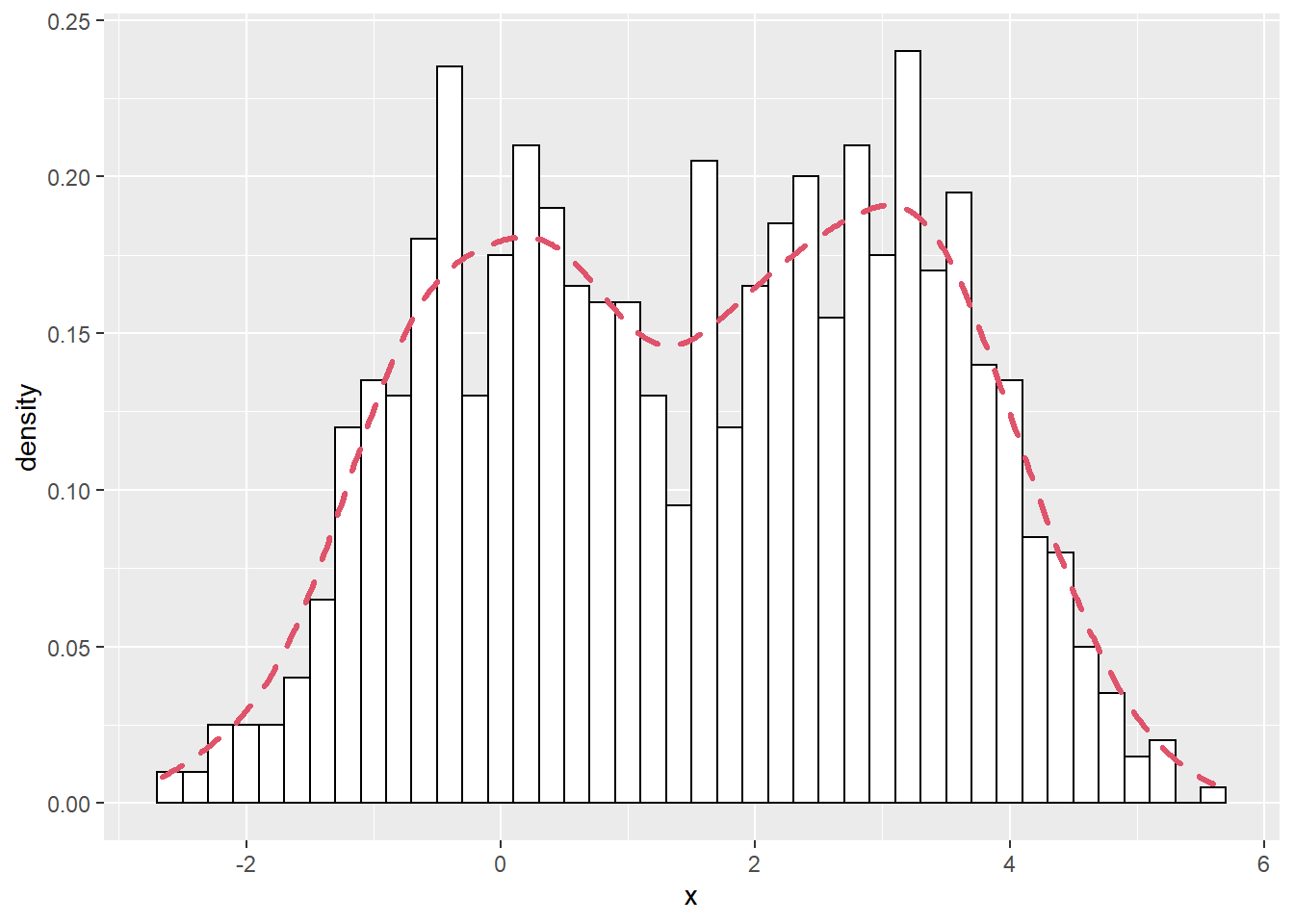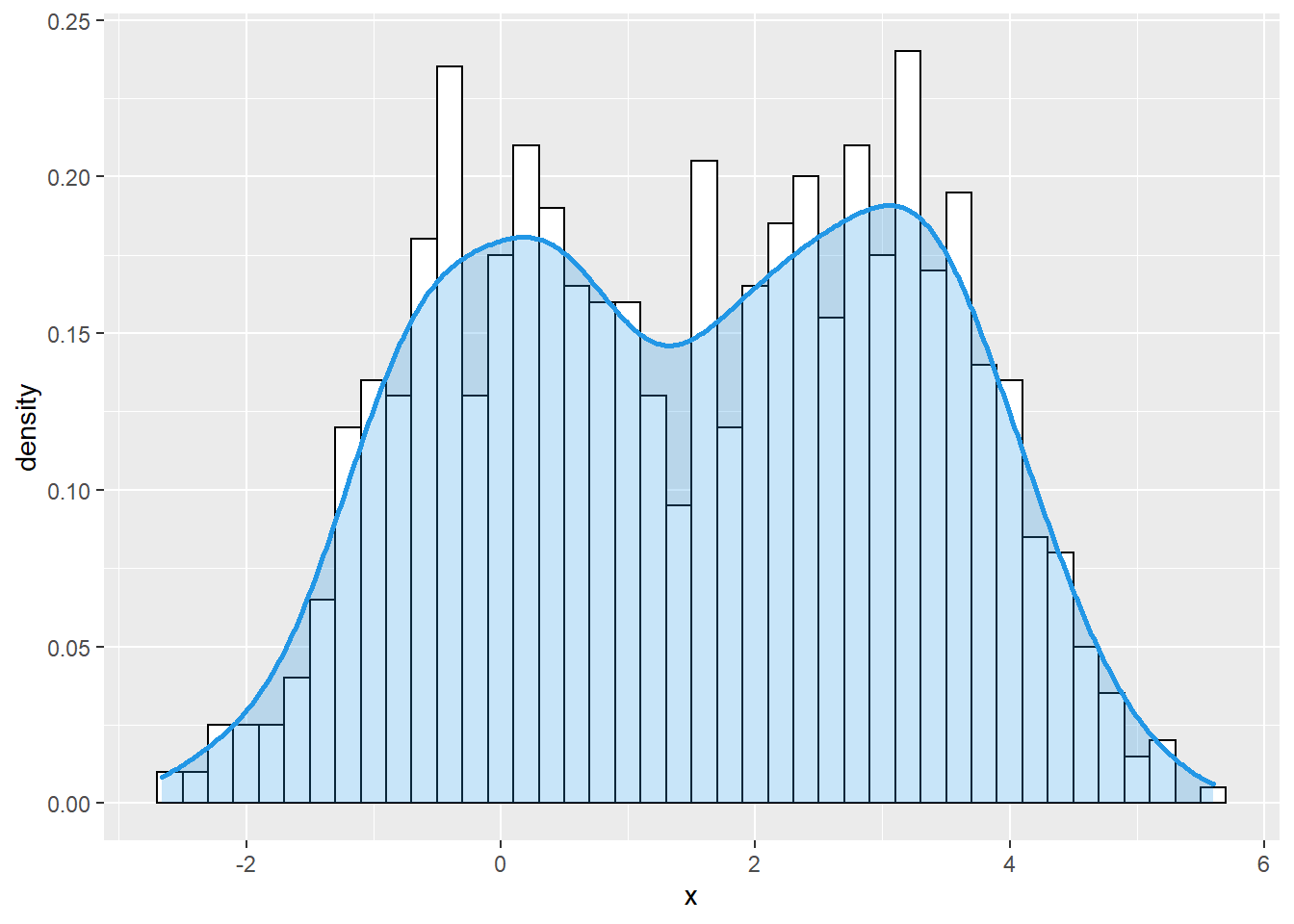## R Data Visualisation Example: Histogram with density using ggplot2

### Import library

library(ggplot2)

### Generate Sample Data for Plotting purposes

# Sample data
set.seed(007)

set.seed(3)
x1 <- rnorm(500)
x2 <- rnorm(500, mean = 3)
x <- c(x1, x2)
group <- c(rep("G1", 500), rep("G2", 500))

df <- data.frame(x, group = group)

head(df)
##             x group
## 1 -0.96193342    G1
## 2 -0.29252572    G1
## 3  0.25878822    G1
## 4 -1.15213189    G1
## 5  0.19578283    G1
## 6  0.03012394    G1

## Basic Histogram with density using ggplot2

### Histogram with kernel density estimation

In order to overlay a kernel density estimate over a histogram in ggplot2 you will need to pass aes(y = ..density..) to geom_histogram and add geom_density as in the example below.

# Histogram with kernel density
ggplot(df, aes(x = x)) +
geom_histogram(aes(y = ..density..),
colour = 1, fill = "white", binwidth = 0.2) +
geom_density()### Curve customization

The color, line width and line type of the kernel density curve can be customized making use of colour, lwd and linetype arguments.

# Histogram with kernel density
ggplot(df, aes(x = x)) +
geom_histogram(aes(y = ..density..),
colour = 1, fill = "white", binwidth = 0.2) +
geom_density(lwd = 1.2,
linetype = 2,
colour = 2)### Density curve with shaded area

You can also shade the area behind the curve, specifying a fill color with the fill argument of the geom_density function. It is recommended to set a level of transparency (between 0 and 1) with alpha argument, so the histogram will keep visible.

# Histogram with kernel density
ggplot(df, aes(x = x)) +
geom_histogram(aes(y = ..density..),
colour = 1, fill = "white", binwidth = 0.2) +
geom_density(lwd = 1, colour = 4,
fill = 4, alpha = 0.25)